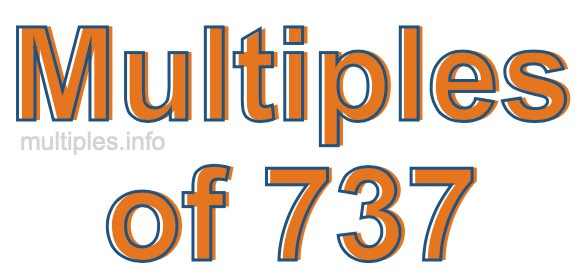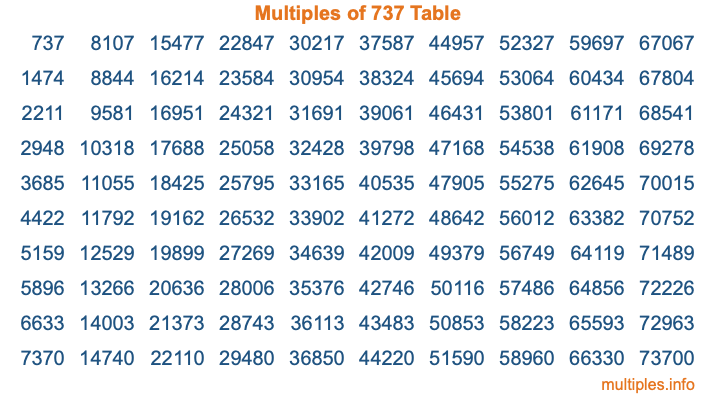Multiples of 737Welcome to the Multiples of 737 page. Here we will first teach you everything you will ever need to know about the multiples of 737, and then give you a study guide summary of everything we taught you to make sure you remember it all. Use this page to look up facts and learn information about the multiples of 737. This page will make you a multiples of seven hundred thirty-seven expert!

Definition of Multiples of 737
Multiples of 737 are all the numbers that when divided by 737 equal an integer. Each of the multiples of 737 are called a multiple. A multiple of 737 is created by multiplying 737 by an integer.

Therefore, to create a list of multiples of 737, you start with 1 multiplied by 737, then 2 multiplied by 737, then 3 multiplied by 737, and so on for as long as you want. Thus, the list of the first five multiples of 737 is 737, 1474, 2211, 2948, and 3685. To see a larger list of multiples of 737, see the printable image of Multiples of 737 further down on this page. We also have a category where you can choose any nth multiple of 737.

Multiples of 737 Checker
The Multiples of 737 Checker below checks to see if any number of your choice is a multiple of 737. In other words, it checks to see if there is any number (integer) that when multiplied by 737 will equal your number. To do that, we divide your number by 737. If the the quotient is an integer, then your number is a multiple of 737.

Is  a multiple of 737?

Least Common Multiple of 737 and ...
A Least Common Multiple (LCM) is the lowest multiple that two or more numbers have in common. This is also called the smallest common multiple or lowest common multiple and is useful to know when you are adding our subtracting fractions. Enter one or more numbers below (737 is already entered) to find the LCM.

Check out our LCM Calculator if you need more details about the Least Common Multiple or if you need the LCM for different numbers for adding and subtraction fractions.

nth Multiple of 737
As we stated above, 737 is the first multiple of 737, 1474 is the second multiple of 737, 2211 is the third multiple of 737, and so on. Enter a number below to find the nth multiple of 737.

th multiple of 737

Multiples of 737 vs Factors of 737
737 is a multiple of 737 and a factor of 737, but that is where the similarities end. All postive multiples of 737 are 737 or greater than 737. All positive factors of 737 are 737 or less than 737.

Below is the beginning list of multiples of 737 and the factors of 737 so you can compare:

Multiples of 737: 737, 1474, 2211, 2948, 3685, etc.

Factors of 737: 1, 11, 67, 737

As you can see, the multiples of 737 are all the numbers that you can divide by 737 to get a whole number. The factors of 737, on the other hand, are all the whole numbers that you can multiply by another whole number to get 737.

It's also interesting to note that if a number (x) is a factor of 737, then 737 will also be a multiple of that number (x).

Multiples of 737 vs Divisors of 737
The divisors of 737 are all the integers that 737 can be divided by evenly. Below is a list of the divisors of 737.

Divisors of 737: 1, 11, 67, 737

The interesting thing to note here is that if you take any multiple of 737 and divide it by a divisor of 737, you will see that the quotient is an integer.

Multiples of 737 Table
Below is an image of the first 100 multiples of 737 in a table. The table is in chronological order, column by column. The first column has the first ten multiples of 737, the second column has the next ten multiples of 737, and so on.The Multiples of 737 Table is also referred to as the 737 Times Table or Times Table of 737. You are welcome to print out our table for your studies.

Negative Multiples of 737
Although not often discussed or needed in math, it is worth mentioning that you can make a list of negative multiples of 737 by multiplying 737 by -1, then by -2, then by -3, and so on, to get the following list of negative multiples of 737:

-737, -1474, -2211, -2948, -3685, etc.

Multiples of 737 Summary
Below is a summary of important Multiples of 737 facts that we have discussed on this page. To retain the knowledge on this page, we recommend that you read through the summary and explain to yourself or a study partner why they hold true.

There are an infinite number of multiples of 737.

A multiple of 737 divided by 737 will equal a whole number.

737 divided by a factor of 737 equals a divisor of 737.

The nth multiple of 737 is n times 737.

The largest factor of 737 is equal to the first positive multiple of 737.

737 is a multiple of every factor of 737.

737 is a multiple of 737.

A multiple of 737 divided by a divisor of 737 equals an integer.

737 divided by a divisor of 737 equals a factor of 737.

Any integer times 737 will equal a multiple of 737.

Multiples of a Number
Here you can get the multiples of another number, all with the same attention to detail as we did for multiples of 737 on this page.

Multiples of
Multiples of 738
Did you find our page about multiples of seven hundred thirty-seven educational? Do you want more knowledge? Check out the multiples of the next number on our list!